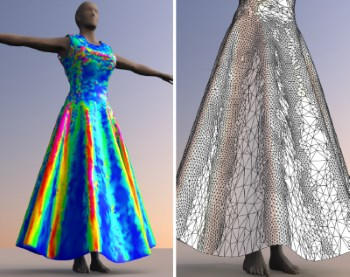# Adaptive cloth simulation using corotational finite elements

Jan Bender, Crispin Deul
Computers & GraphicsIn this article we introduce an efficient adaptive cloth simulation method which is based on a reversible $\sqrt{3}$-refinement of corotational finite elements. Our novel approach can handle arbitrary triangle meshes and is not restricted to regular grid meshes which are required by other adaptive methods. Most previous works in the area of adaptive cloth simulation use discrete cloth models like mass-spring systems in combination with a specific subdivision scheme. However, if discrete models are used, the simulation does not converge to the correct solution as the mesh is refined. Therefore, we introduce a cloth model which is based on continuum mechanics since continuous models do not have this problem. We use a linear elasticity model in combination with a corotational formulation to achieve a high performance. Furthermore, we present an efficient method to update the sparse matrix structure after a refinement or coarsening step. The advantage of the $\sqrt{3}$-subdivision scheme is that it generates high quality meshes while the number of triangles increases only by a factor of 3 in each refinement step. However, the original scheme was not intended for the use in an interactive simulation and only defines a mesh refinement. In this article we introduce a combination of the original refinement scheme with a novel coarsening method to realize an adaptive cloth simulation with high quality meshes. The proposed approach allows an efficient mesh adaption and therefore does not cause much overhead. We demonstrate the significant performance gain which can be achieved with our adaptive simulation method in several experiments including a complex garment simulation.

» Show BibTeX

@ARTICLE{Bender2013,
author = {Jan Bender and Crispin Deul},
title = {Adaptive cloth simulation using corotational finite elements },
journal = {Computers \& Graphics },
year = {2013},
volume = {37},
pages = {820 - 829},
number = {7},
doi = {http://dx.doi.org/10.1016/j.cag.2013.04.008},
url = {http://www.sciencedirect.com/science/article/pii/S0097849313000605},
issn = {0097-8493}
}